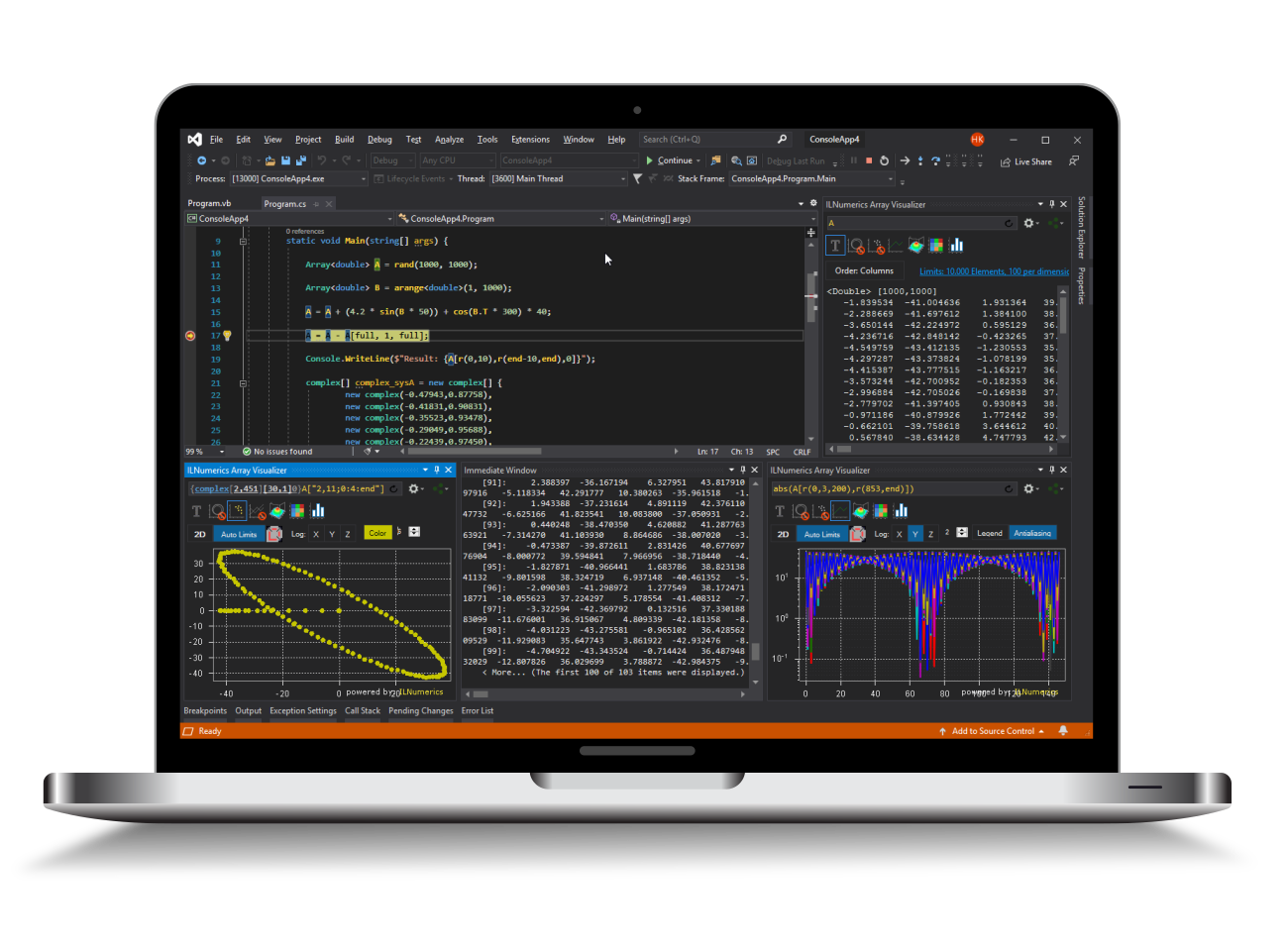Industrial Data Science
in C# and .NET:
Simple. Fast. Reliable.ILNumerics - Technical Computing

Modern High Performance Tools for Technical

Computing and Visualization in Industry and Science

tgt

# Statistics Toolbox for .NET (C# and Visual Basic)

ILNumerics offers a range of statistical functions and distributions to extend the computing capability out of the box. Click on the name of a function in order to go to the corresponding API documentation.

Method name Short description
Statistics Functions
cov Calculates the covariance matrix or variance of a vector.
median Calculates median along the specified dimension of an array.

mvnpdf

Calculates the probability density function for a multivariate normal random distribution.
mvnrnd Chooses samples from a multivariate random distribution.
randperm Creates a random permutation of integers.
std Calculates the standard deviation for values in an array.
var Calculates the variance along the specified dimension of an array.
nansum Sums the elements along first non singleton dimension ignoring NaN values.
nanmean Mean of array along specified dimension ignoring NaN values.
hist Calculates the histogram of the given samples.
histn Calculates the normalized (with the number of the samples) histogram of the given samples.
histc Calculates the cumulated histogram of the given samples.
histnc Calculates the normalized (with the number of the samples) cumulated histogram of the given samples.

Furthermore, the following Distributions are offered as unique classes, with their methods and properties:

Distribution Short description
Distributions
Chi Continuous Univariate Chi distribution.
ChiSquare Continuous Univariate Chi-Squared distribution.
Hypergeometric Discrete Univariate Hypergeometric distribution.

LogNormal

Continuous Univariate Log-Normal distribution.
Normal Normal or Gaussian distribution.
Student Continuous Univariate Student's T-distribution.